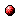# The characteristic equation and minimal state space realization of SISO systems in the max algebra

Reference:
B. De Schutter and B. De Moor, "The characteristic equation and minimal state space realization of SISO systems in the max algebra," in 11th International Conference on Analysis and Optimization of Systems (Sophia-Antipolis, France, June 1994) (G. Cohen and J.P. Quadrat, eds.), vol. 199 of Lecture Notes in Control and Information Sciences, Springer, ISBN 3-540-19896-2, pp. 273-282, 1994.

Abstract:
In this paper we determine necessary and for some cases also sufficient conditions for a polynomial to be the characteristic polynomial of a matrix with elements in Rmax. Then we indicate how to construct a matrix such that its characteristic polynomial is equal to a given monic polynomial in Smax, the extension of Rmax. We use these results to develop a procedure to find a minimal state space realization of a single input single output (SISO) discrete event system in the max algebra, given its Markov parameters.Online version of the paperCorresponding technical report: pdf file (153 KB)
Note: More information on the pdf file format mentioned above can be found here.

Bibtex entry:

@incollection{DeSDeM:93-57a,
author={B. {D}e Schutter and B. {D}e Moor},
title={The characteristic equation and minimal state space realization of {SISO} systems in the max algebra},
booktitle={11th International Conference on Analysis and Optimization of Systems \rm(Sophia-Antipolis, France, June 1994)},
series={Lecture Notes in Control and Information Sciences},
volume={199},